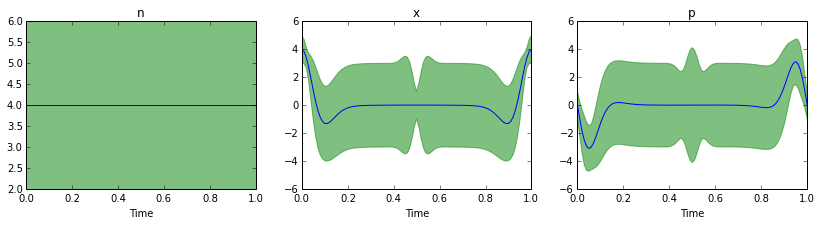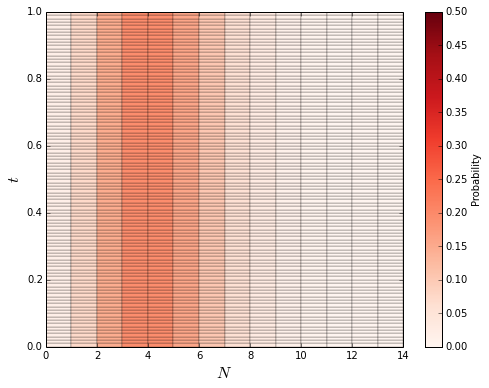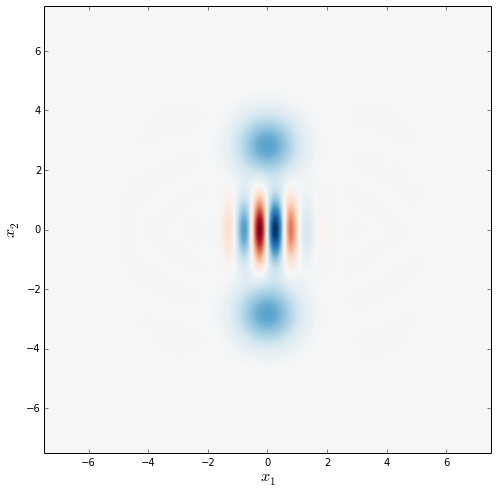# Lecture 14: Kerr nonlinearities¶

Author: J.R. Johansson, [email protected]

http://jrjohansson.github.io

In :
%matplotlib inline
import matplotlib as mpl
import matplotlib.pyplot as plt
from numpy import *

In :
from IPython.display import HTML

In :
from matplotlib import animation

In :
from qutip import *


## Introduction¶

The Kerr effect describes a self-interaction electromagnetic quantum field which occur in a nonlinear medium. A single mode of the quantum field can be described by the effective Hamiltonian

$\displaystyle H = \frac{1}{2}\chi (a^\dagger)^2a^2$

where $\chi$ is related to the third-order nonlinear suseptibility. The Kerr effect is one of the typical nonlinearities that can occur in quantum optics due to a nonlinear medium.

In this notebook we'll see how to setup the model in QuTiP and look at some interesting properties of the states that evolve according to this Hamiltonian.

## Parameters¶

In :
N = 15
chi = 1 * 2 * pi              # Kerr-nonlinearity
tlist = linspace(0, 1.0, 101) # time

In :
# operators: the annihilation operator of the field
a = destroy(N)

# and we'll also need the following operators in calculation of
# expectation values when visualizing the dynamics
n = num(N)
x = a + a.dag()
p = -1j * (a - a.dag())

In :
# the Kerr Hamiltonian
H = 0.5 * chi * a.dag() * a.dag() * a * a


## Functions for plotting¶

We start by defining some function for visualizing the dynamics. We'll use these further down in the notebook.

In :
def plot_expect_with_variance(N, op_list, op_title, states):
"""
Plot the expectation value of an operator (list of operators)
with an envelope that describes the operators variance.
"""

fig, axes = plt.subplots(1, len(op_list), figsize=(14,3))

for idx, op in enumerate(op_list):

e_op = expect(op, states)
v_op = variance(op, states)

axes[idx].fill_between(tlist, e_op - sqrt(v_op), e_op + sqrt(v_op), color="green", alpha=0.5);
axes[idx].plot(tlist, e_op)
axes[idx].set_xlabel('Time')
axes[idx].set_title(op_title[idx])
axes[idx].set_xlim(0, max(tlist))

return fig, axes

In :
def plot_wigner(rho, fig=None, ax=None):
"""
Plot the Wigner function and the Fock state distribution given a density matrix for
a harmonic oscillator mode.
"""

if fig is None or ax is None:
fig, ax = plt.subplots(1, 1, figsize=(8,8))

if isket(rho):
rho = ket2dm(rho)

xvec = linspace(-7.5,7.5,200)

W = wigner(rho, xvec, xvec)
wlim = abs(W).max()

ax.contourf(xvec, xvec, W, 100, norm=mpl.colors.Normalize(-wlim,wlim), cmap=mpl.cm.get_cmap('RdBu'))
ax.set_xlabel(r'$x_1$', fontsize=16)
ax.set_ylabel(r'$x_2$', fontsize=16)

return fig, ax

In :
def plot_fock_distribution_vs_time(tlist, states, fig=None, ax=None):

Z = zeros((len(tlist), states.shape))

for state_idx, state in enumerate(states):
Z[state_idx,:] = real(ket2dm(state).diag())

if fig is None or axes is None:
fig, ax = plt.subplots(1, 1, figsize=(8,6))

Y, X = meshgrid(tlist, range(states.shape))
p = ax.pcolor(X, Y, Z.T, norm=mpl.colors.Normalize(0, 0.5), cmap=mpl.cm.get_cmap('Reds'), edgecolors='k')
ax.set_xlabel(r'$N$', fontsize=16)
ax.set_ylabel(r'$t$', fontsize=16)

cb = fig.colorbar(p)
cb.set_label('Probability')

return fig, ax

In :
from base64 import b64encode

def display_embedded_video(filename):
video_encoded = b64encode(video).decode("ascii")
video_tag = '<video controls alt="test" src="data:video/x-m4v;base64,{0}">'.format(video_encoded)
return HTML(video_tag)


## Coherent state¶

Let's look at how the coherent state evolves under the influence of the Kerr Hamiltonian.

In :
# we start with a coherent state with alpha=2.0
psi0 = coherent(N, 2.0)

In :
# and evolve the state under the influence of the hamiltonian.
# by passing an empty list as expecation value operators argument,
# we get the full state of the system in result.states
result = mesolve(H, psi0, tlist, [], [])


First, let's look at how the expecation values and variances of the photon number operator $n$ and the $x$ and $p$ quadratures evolve in time:

In :
plot_expect_with_variance(N, [n, x, p], [r'n', r'x', r'p'], result.states);Note that the average photon number $\langle n \rangle$ and the corresponding variance is constant, suggesting that the Fock state distribution is preserved. From the plots above we can also see that during the evolution the variance in $x$ and $p$ change as a function of time.

To verify that the photon distribution indeed is time-independent, we can plot the fock distribution as a function of time:

In :
plot_fock_distribution_vs_time(tlist, result.states);So the fock state distribution is constant, but let's see how the Wigner function of the state evolves in time. To best illustrate the dynamics of the Winger function we make a short movie that show the Wigner function from time $t=0$ to the the final time of the evolution.

In :
fig, ax = plt.subplots(1, 1, figsize=(8,8))

def update(n):
plot_wigner(result.states[n], fig=fig, ax=ax)

anim = animation.FuncAnimation(fig, update, frames=len(result.states), blit=True)

anim.save('animation-kerr-coherent-state.mp4', fps=10, writer="avconv", codec="libx264")
#anim.save('animation-kerr-coherent-state.gif', writer='imagemagick', fps=10)

plt.close(fig)

In :
display_embedded_video("animation-kerr-coherent-state.mp4")

Out:

Isn't that interesting! The dynamics is periodic, and we evolved the state for exactly one period, so that the final state is equal to the initial state.

In between there is interesting stuff going on. For example, after half the period the state ends up in something that look very much like a cat-state superposition of coherent states!

In :
fig, ax = plt.subplots(1, 1, figsize=(8, 8))

def update(n):
plot_wigner(result.states[n], fig=fig, ax=ax)

anim = animation.FuncAnimation(fig, update, frames=int(len(result.states)/2+1), blit=True)

#anim.save('animation-kerr-coherent-state-half-period.mp4', fps=10, extra_args=['-vcodec', 'libx264'])
anim.save('animation-kerr-coherent-state-half-period.mp4', fps=10, writer="avconv", codec="libx264")

plt.close(fig)

In :
display_embedded_video('animation-kerr-coherent-state-half-period.mp4')

Out:

And indeed, evolving a coherent state $|\alpha\rangle$ for the time $\chi t = \pi$ results in the cat state

$\psi = \frac{1}{\sqrt{2}}\left(e^{i\pi/4}|-i\alpha\rangle + e^{-i\pi/4}|i\alpha\rangle\right)$

(See Walls and Milburn, Quantum Optics, p91)

In :
psi = (exp(1j*pi/4) * coherent(N, -2.0j) + exp(-1j*pi/4) * coherent(N, 2.0j)).unit()

In :
plot_wigner(psi);### Software versions¶

In :
from qutip.ipynbtools import version_table

version_table()

Out:
SoftwareVersion
Cython0.20.1
SciPy0.14.0
QuTiP3.0.0.dev-f019caa
Python2.7.9 (default, Dec 13 2014, 15:13:49) [GCC 4.2.1 Compatible Apple LLVM 6.0 (clang-600.0.56)]
IPython2.1.0
OSposix [darwin]
Numpy1.9.0
matplotlib1.3.1
Fri Jan 23 12:14:19 2015 JST

### Acknowledgements¶

Thanks Sander Konijnenberg for correcting an error in the analytical expression for the Cat state.